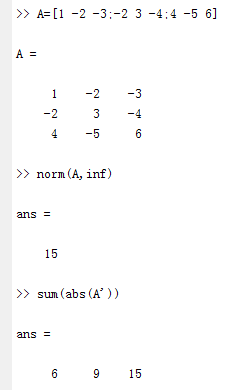# MATLAB中范数norm()函数精讲

## 1、Matlab中norm有两种形式：

1.n = norm(A)
2.n = norm(A,p) ，p – 范数

A可以是向量、也可以是矩阵；根据p的不同，norm函数可计算几种不同类型的矩阵（向量）范数，1<p<+∞。

## 2、若A为向量（向量范数）

### 2.1 格式：

norm(A,p)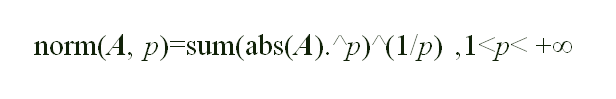1范数：为所有元素绝对值之和，即sum(abs(A))
2范数：就是通常意义上的模，即sqrt(sum(abs(A.^2)))

### 2.2 举例

eg：以向量A=[0 -1 2 -3 4]为例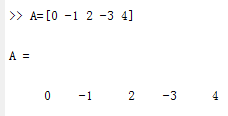#### 2.2.1 1范数：所有元素绝对值之和,即sum(abs(A))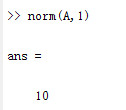#### 2.2.2 2范数：通常意义上的模，欧几里德范数，谱范数，即sqrt(sum(abs(A.^2)))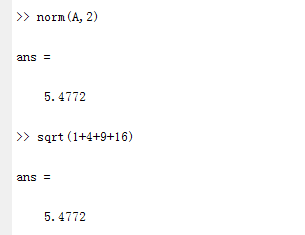#### 2.2.3 无穷范数(inf)：取向量中所有元素绝对值的最大值，即max(abs(A))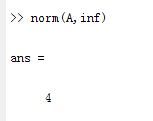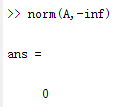## 3、若A为矩阵（矩阵范数）

### 3.1 格式：1范数：返回矩阵A中最大一列和，即max(sum(abs(A))) 。
2范数：就是通常意义上的模，即返回的是矩阵A的二范数，(二范数j就是矩阵A的2范数就是 A的转置矩阵乘以A特征根 最大值的开根号)

### 3.2 举例：

eg：以矩阵A=[1 -2 -3;-2 3 -4;4 -5 6]为例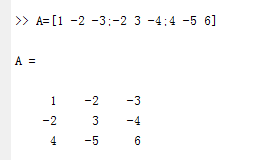#### 3.2.1 1范数：返回A中元素绝对值最大一列和，即norm(A,1)=max(sum(abs(A))) ，其中sum(abs(A))表示每列元素绝对值之和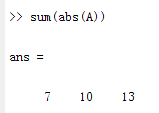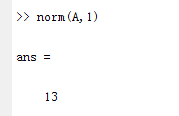#### 3.2.2 2范数：返回的是矩阵A的最大奇异值，指矩阵A与矩阵A的转置相乘后得到B，再对矩阵B的最大特征值开方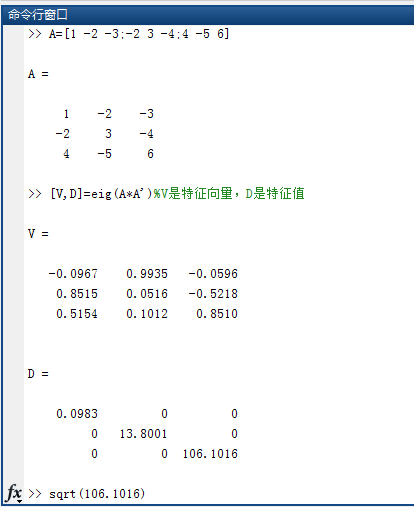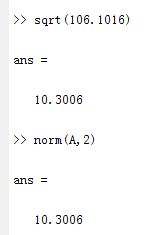#### 3.2.3 无穷范数(inf)：返回A中元素绝对值最大一行和，即norm(A,inf)=max(sum(abs(A‘))) ，其中sum(abs(A’))表示一行元素绝对值之和